Updating search results...

# 13 Results

View
Selected filters:
• MCCRS.Math.Content.7.EE.A.2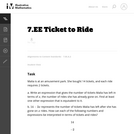Unrestricted Use
CC BY
Rating
0.0 stars

This is a task from the Illustrative Mathematics website that is one part of a complete illustration of the standard to which it is aligned. Each task has at least one solution and some commentary that addresses important asects of the task and its potential use. Here are the first few lines of the commentary for this task: Malia is at an amusement park. She bought 14 tickets, and each ride requires 2 tickets. Write an expression that gives the number of tickets Malia has ...

Subject:
Mathematics
Material Type:
Activity/Lab
Provider:
Illustrative Mathematics
Provider Set:
Illustrative Mathematics
Author:
Illustrative Mathematics
09/08/2013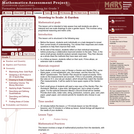Only Sharing Permitted
CC BY-NC-ND
Rating
0.0 stars

This lesson unit is intended to help assess how well students are able to interpret and use scale drawings to plan a garden layout. This involves using proportional reasoning and metric units.

Subject:
Algebra
Geometry
Mathematics
Ratios and Proportions
Material Type:
Assessment
Lesson Plan
Provider:
Shell Center for Mathematical Education
Provider Set:
Mathematics Assessment Project (MAP)
04/26/2013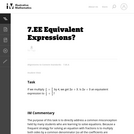Unrestricted Use
CC BY
Rating
0.0 stars

The purpose of this task is to directly address a common misconception held by many students who are learning to solve equations. Because a frequent strategy for solving an equation with fractions is to multiply both sides by a common denominator (so all the coefficients are integers), students often forget why this is an "allowable" move in an equation and try to apply the same strategy when they see an expression.

Subject:
Algebra
Mathematics
Material Type:
Activity/Lab
Provider:
Illustrative Mathematics
Provider Set:
Illustrative Mathematics
Author:
Illustrative Mathematics
05/01/2012Conditional Remix & Share Permitted
CC BY-NC
Rating
0.0 stars

Four full-year digital course, built from the ground up and fully-aligned to the Common Core State Standards, for 7th grade Mathematics. Created using research-based approaches to teaching and learning, the Open Access Common Core Course for Mathematics&nbsp;is designed with student-centered learning in mind, including activities for students to develop valuable 21st century skills and academic mindset.

Subject:
Mathematics
Material Type:
Full Course
Provider:
Pearson
10/06/2016Conditional Remix & Share Permitted
CC BY-NC
Rating
0.0 stars

Algebraic Reasoning

Type of Unit: Concept

Prior Knowledge

Students should be able to:

Add, subtract, multiply, and divide rational numbers.
Evaluate expressions for a value of a variable.
Use the distributive property to generate equivalent expressions including combining like terms.
Understand solving an equation or inequality as a process of answering a question: which values from a specified set, if any, make the equation or inequality true?
Write and solve equations of the form x+p=q and px=q for cases in which p, q, and x are non-negative rational numbers.
Understand and graph solutions to inequalities x&lt;c or x&gt;c.
Use equations, tables, and graphs to represent the relationship between two variables.
Relate fractions, decimals, and percents.
Solve percent problems included those involving percent of increase or percent of decrease.

Lesson Flow

This unit covers all of the Common Core State Standards for Expressions and Equations in Grade 7. Students extend what they learned in Grade 6 about evaluating expressions and using properties to write equivalent expressions. They write, evaluate, and simplify expressions that now contain both positive and negative rational numbers. They write algebraic expressions for problem situations and discuss how different equivalent expressions can be used to represent different ways of solving the same problem. They make connections between various forms of rational numbers. Students apply what they learned in Grade 6 about solving equations such as x+2=6 or 3x=12 to solving equations such as 3x+6=12 and 3(x−2)=12. Students solve these equations using formal algebraic methods. The numbers in these equations can now be rational numbers. They use estimation and mental math to estimate solutions. They learn how solving linear inequalities differs from solving linear equations and then they solve and graph linear inequalities such as −3x+4&lt;12. Students use inequalities to solve real-world problems, solving the problem first by arithmetic and then by writing and solving an inequality. They see that the solution of the algebraic inequality may differ from the solution to the problem.

Subject:
Algebra
Mathematics
Material Type:
Unit of Study
Provider:
Pearson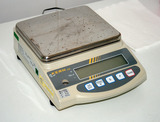Conditional Remix & Share Permitted
CC BY-NC
Rating
0.0 stars

Students use algebraic expressions and equations to represent rules of thumb involving measurement. They use properties of operations and the relationships between fractions, decimals, and percents to write equivalent expressions.Key ConceptsExpressions and equations are different. An expression is a number, a variable, or a combination of numbers and variables. Some examples of expressions are:74x5a + b3(2m + 1)In Grade 7, the focus is on linear expressions. A linear expression is a sum of terms that are either rational numbers or a rational number times a variable (with an exponent of either 0 or 1). If an expression contains a variable, it is called an algebraic expression. To evaluate an expression, each variable is replaced with a given value.Equivalent expressions are expressions for which a given value can be substituted for each variable and the value of the expressions are the same.An equation is a statement that two expressions are equal. An equation can be true or false. To solve an equation, students find the value of the variable that makes the equation true.Students solve an equation that involves finding 10% of a number. They see that finding 10% of the number is the same as finding 0.1 of the number, or finding 110 of the number.Goals and Learning ObjectivesWrite expressions and equations to represent real-world situations.Evaluate expressions for given values of a variable.Use properties of operations to write equivalent expressions.Solve one-step equations.Check the solution to an equation.

Subject:
Algebra
Material Type:
Lesson Plan
09/21/2015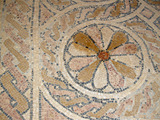Conditional Remix & Share Permitted
CC BY-NC
Rating
0.0 stars

Students write expressions for geometric situations. They examine how different equivalent expressions can show different ways of thinking about the same problem.Key ConceptsStudents use their previous knowledge of how to find the perimeter and area of squares and rectangles. They write algebraic expressions for the perimeter and area of geometric figures. They examine how equivalent expressions, used to represent a problem situation, give clues to the approach the writer of the expression used to solve the problem. In the Challenge Problem, they use the distributive property to find the solution.ELL: For ELLs, access prior knowledge by writing the words area and perimeter on the board. Have students create concept maps associated with area and perimeter. Record students&#39; responses on large poster paper that you can display in the room. The goal is to generate a list of words that students can use as a reference.Goals and Learning ObjectivesAccess prior knowledge of how to find the perimeter and area of squares and rectangles.Write algebraic expressions for finding perimeter or area of figures.Identify equivalent expressions.

Subject:
Geometry
Material Type:
Lesson Plan
09/21/2015Conditional Remix & Share Permitted
CC BY-NC
Rating
0.0 stars

Students use the distributive property to simplify expressions. Simplifying expressions may include multiplying by a negative number. Students analyze and identify errors that are sometimes made when simplifying expressions.Key ConceptsThis lesson focuses on simplifying expressions and requires an understanding of the rules for multiplying negative numbers. For example, students simplify expressions such as 8 &minus; 3(2 &minus; 4x). These kinds of expressions are often difficult for students because there are several errors that they can make based on misconceptions:Students may simplify 8 &minus; 3(2 &minus; 4x) to 5(2 &minus; 4x) because they mistakenly detach the 3 from the multiplication.Students may simplify 8 &minus; 3(2 &minus; 4x) to 8 &minus; 3(&minus;2x) in an attempt to simplify the expression in parentheses even though no simplification is possible.Students may simplify 8 &minus; 3(2 &minus; 4x) to 8 &minus; 6 &minus;12x. This error could be based on a misunderstanding of how the distributive property works or on lack of knowledge of the rules for multiplying integers.Goals and Learning ObjectivesSimplify more complicated expressions that involve multiplication by negative numbers.Identify errors that can be made when simplifying expressions.

Subject:
Algebra
Material Type:
Lesson Plan
09/21/2015Conditional Remix & Share Permitted
CC BY-NC
Rating
0.0 stars

Students see how different expressions for percent of increase and percent of decrease problems represent different ways to solve these problems. Students use equivalent algebraic expressions to solve percent problems.Key ConceptsStudents have previously solved percent of increase and percent of decrease problems. In this lesson, they look at how percent problems can be represented by algebraic expressions. Seeing the relationship of these problems to various equivalent algebraic expressions helps students relate different ways of solving problems involving percent of increase or percent of decrease.For example, the sale price of a pair of jeans with original price p and discount of 10% can be represented as&nbsp;p &minus; 0.1p, or just 0.9p. The first expression leads to a way of solving the problem in two steps; the second expression leads to a one-step solution. Similarly, the total price of an item with a cost c dollars and 5% tax can be written as c + 0.05c, or just 1.05c.Goals and Learning ObjectivesSolve percent of increase and percent of decrease problems using equivalent algebraic expressions.

Subject:
Algebra
Material Type:
Lesson Plan
09/21/2015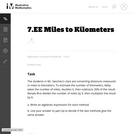Unrestricted Use
CC BY
Rating
0.0 stars

In this task students are asked to write two expressions from verbal descriptions and determine if they are equivalent. The expressions involve both percent and fractions. This task is most appropriate for a classroom discussion since the statement of the problem has some ambiguity.

Subject:
Algebra
Mathematics
Material Type:
Activity/Lab
Provider:
Illustrative Mathematics
Provider Set:
Illustrative Mathematics
Author:
Illustrative Mathematics
05/01/2012Educational Use
Rating
0.0 stars

Waterwheels are devices that generate power and do work. Student teams construct waterwheels using two-liter plastic bottles, dowel rods and index cards, and calculate the power created and work done by them.

Subject:
Applied Science
Engineering
Material Type:
Activity/Lab
Provider:
TeachEngineering
Provider Set:
TeachEngineering
Author:
Bailey Jones
Chris Yakacki
Denise W. Carlson
Malinda Schaefer Zarske
Matt Lundberg
10/14/2015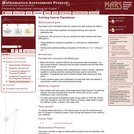Only Sharing Permitted
CC BY-NC-ND
Rating
0.0 stars

This lesson unit is intended to help teachers assess how well students are able to: form and solve linear equations involving factorizing and using the distributive law. In particular, this unit aims to help teachers identify and assist students who have difficulties in: using variables to represent quantities in a real-world or mathematical problem and solving word problems leading to equations of the form px + q = r and p(x + q) = r.

Subject:
Algebra
Mathematics
Material Type:
Assessment
Lesson Plan
Provider:
Shell Center for Mathematical Education
Provider Set:
Mathematics Assessment Project (MAP)
04/26/2013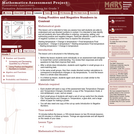Only Sharing Permitted
CC BY-NC-ND
Rating
0.0 stars

This lesson unit is intended to help teachers assess how well students are able to understand and use directed numbers in context. It is intended to help identify and aid students who have difficulties in ordering, comparing, adding, and subtracting positive and negative integers. Particular attention is paid to the use of negative numbers on number lines to explore the structures: starting temperature + change in temperature = final temperature final temperature Đ change in temperature = starting temperature final temperature Đ starting temperature = change in temperature.

Subject:
Mathematics
Numbers and Operations
Material Type:
Assessment
Lesson Plan
Provider:
Shell Center for Mathematical Education
Provider Set:
Mathematics Assessment Project (MAP)